# Thermal Expansion

Over small temperature ranges, the linear nature of thermal expansion leads to expansion relationships for length, area, and volume in terms of the linear expansion coefficient .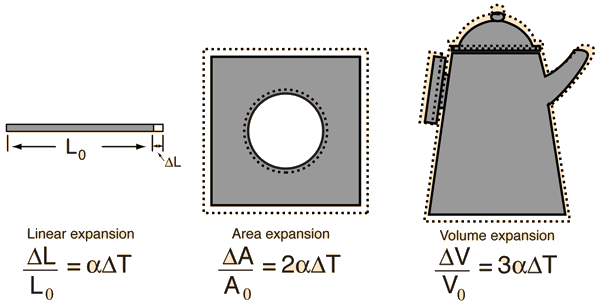Table of expansion coefficients
Index

Expansion concepts

 HyperPhysics***** Thermodynamics R Nave
Go Back

# Linear Expansion

The relationship governing the linear expansion of a long thin rod can be reasoned out as follows: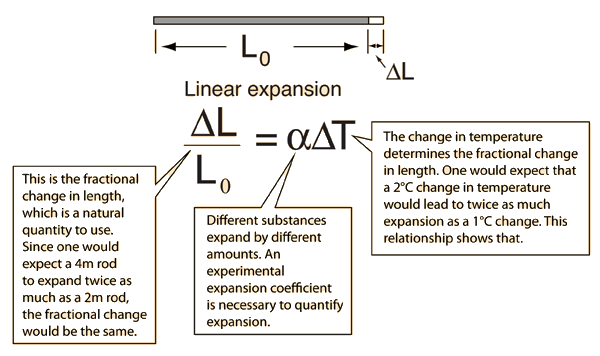Expansion concepts Calculation
Index

 HyperPhysics***** Thermodynamics R Nave
Go Back

# Linear Expansion

Over small temperature ranges, the fractional thermal expansion of uniform linear objects is proportional the the temperature change. This fact can be used to construct thermometers based on the expansion of a thin tube of mercury or alcohol. Several equivalent forms of the relationship find use. The calculation is set up in the form

### Length change = Original length x alpha x delta T

 Common forms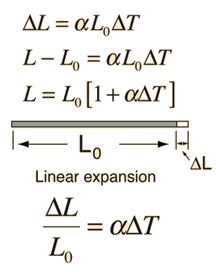A length change ΔL = m will occur for an object of original length L0 = m and expansion coefficient = x10^ /C. Original temperature = C = F Final temperature = C = F Note: This calculation is set up with default values corresponding to heating a 10 meter bar of steel by 20 °C. Any of the values may be changed. The final calculation is done by clicking on the quantity you wish to calculate in the active expression above.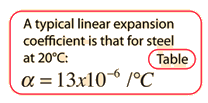Index

Alcohol thermometer Wiki

 HyperPhysics***** Thermodynamics R Nave
Go Back

# Expansion Concepts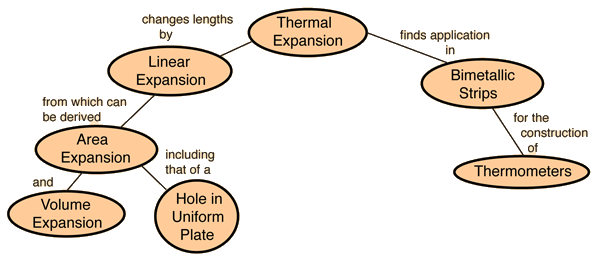How to loosen a jar lid
Index

 HyperPhysics***** Thermodynamics R Nave
Go Back